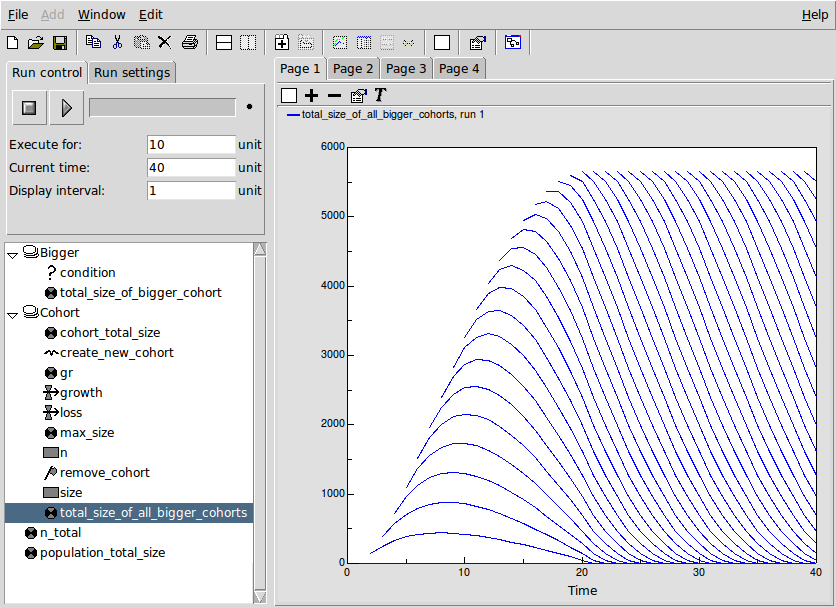# Single-species population dynamics

## Skeleton cohort model

ModelId:
cohort1
SimileVersion:
5.97

This example shows how to implement a basic cohort model in Simile.

A cohort model is one way of modelling a population. The individuals are grouped into cohorts, typically on the basis that they were born at the same time. Each cohort has as a minimum a variable representing the number of individuals in that cohort, along with possibly other cohort-specific attributes, such as the average size of the individuals in the cohort.

Equations:

Variable: n_total = sum({n})

Where: {n} = Cohort/n

Variable: population_total_size = sum({cohort_total_size})
Where: {cohort_total_size} = Cohort/cohort_total_size

--------------------------------------------------------------
Submodel Bigger

Submodel "Bigger" is an association submodel between "Cohort" and itself with roles "other" and "me".

Condition: condition = other_size>me_size
Where:
me_size = Value(s) of ../Cohort/size from submodel "Cohort" in role "me"
other_size = Value(s) of ../Cohort/size from submodel "Cohort" in role "other"

Variable: total_size_of_bigger_cohort = other_cohort_total_size
Where:
me_cohort_total_size = Value(s) of ../Cohort/cohort_total_size from submodel "Cohort" in role "me"
other_cohort_total_size = Value(s) of ../Cohort/cohort_total_size from submodel "Cohort" in role "other"

-------------------------------------------------------------------------
Submodel Cohort

Submodel "Cohort" is a population submodel.

Compartment: n
Initial value = 100
Rate of change = - loss

Compartment: size
Initial value = 0
Rate of change = + growth

Flow: growth = gr*(1-size/max_size)

Flow: loss = 5

Immigration: create_new_cohort = 1

Loss: remove_cohort = n<=0

Variable: cohort_total_size = n*size

Variable: gr = 1.5

Variable: max_size = 10

Variable: total_size_of_all_bigger_cohorts = sum({cohort_total_size_of_bigger_me})
Where:
{cohort_total_size_of_bigger_me} = ../Bigger/total_size_of_bigger_cohort for submodel "Cohort" in role "me"

Results:

The results shown here illustrate the basic functioning of this model.

The following figure shows a set of panels, each one showing the values for a specified variable at time 10. These were made using Simile's 'snapshot' tool, and enable us to trace the values as they are processed in the model.The first 3 panels on the top row show, for each of the 10 cohorts created up to this time, the number of tress (n) and the size of the average tree (size) in the cohort, along with the total size (n x size). You can see that the number of tress declines in a cohort declines by 5 each time step, and that the average tree in a cohort grows at a reducing rate as the cohort gets older.

The bottom window shows the total_size values for the individual cohorts that are bigger than the specified cohort. Thus, cohort 2 only has one value (since there is only one bigger than it is), while cohort 3 has 2 values, and so on.

The top-right window shows 'total_size_of_all_bigger_cohorts' - the sum (along a row) for al the total_size values of bigger cohorts for a particular cohort. This is the key value which enables the impact of bigger cohorts on the growth of a cohort to be modelled.

The graph below shows how the 'total_size_of_all_bigger_cohorts' changes over time, for each cohort. The lowest curve is for the second cohort (since the first cohort has nothing bigger than it. Each successive cohort starts off at a higher value (since the total size of cohorts above it is increasing), until we get to the point where each newly-created cohort starts off with the same total_size above it, which decreases as that cohort in turn increases in size.## Population dynamics: single species: chaotic

ModelId:
chaos
SimileVersion:
5.x

This version differs from the standard population model in two respects:

Equations:

Compartment level

Initial value = rand_const(50,50.000001)
Rate of change = + change

Flow change
change = gain*level*(1-level/100)

﻿

Results:

This graph shows the results of four consecutive runs with gain set to 1.4, 1.9, 2.3 and 2.7 respectively. On the first two runs the level approaches equilibrium; on the third it oscillates around the equilibrium, and on the final run it shows chaotic behaviour. Other behaviours such as period doubling will be shown for other values of gain.This graph illustrates the unpredictability of chaotic behaviour. The model includes a very small random variation in the initial value of level, but the values for three consecutive runs, all with gain set to 2.7, diverge after about 60 time units. Until this time, the plot for the last run (green) exactly overwrites the other two.